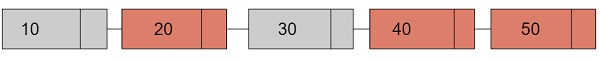# Product of all prime nodes in a Singly Linked List in C++

Given with n nodes and the task is to print the product of all the prime nodes in a linked list. Prime nodes are the ones that will have prime values as their count locations.

Input

10 20 30 40 50

Output

4,00,000

Explanation − 10 is at index value 1 which is non-prime so it will be skipped. Moving to 20 with index value 2 which is a prime number so it will be considered. Similarly, 40 and 50 are at prime index locations.

Product − 20*40*50 = 4,00,000In the above diagram, red coloured nodes represents the prime nodes

## Approach used below is as follows

• Take a temporary pointer, lets say, temp of type node

• Set this temp pointer to first node which is pointed by head pointer

• Move temp to temp→next and check whether the node is a prime node or a non prime node. If the node is a prime node

• DO Set product=product*(temp→data)

• If the node is not prime than move to next node

• Print the final value of product variable.

## Algorithm

Start
Step 1 → create structure of a node to insert into a list
struct node
int data;
node* next
End
Step 2 → declare function to insert a node in a list
Set node* newnode = (node*)malloc(sizeof(struct node))
Set newnode→data = data
End
Step 3 → Declare a function to check for prime or not
bool isPrime(int data)
IF data <= 1
return false
End
IF data <= 3
return true
End
IF data % 2 = 0 || data % 3 = 0
return false
Loop For int i = 5 and i * i <= data and i = i + 6
IFdata % i = 0 || data % (i + 2) = 0
return false
End
End
return true
Step 4→ declare a function to calculate product
set int product = 1
While ptr != NULL
IF (isPrime(ptr→data))
Set product *= ptr→data
End
Set ptr = ptr→next
End
Print product
Step 5 → In main()
Stop

## Example

Live Demo

#include <bits/stdc++.h>
using namespace std;
//structure of a node
struct node{
int data;
node* next;
};
//function to insert a node
node* newnode = (node*)malloc(sizeof(struct node));
newnode→data = data;
}
// Function to check if a number is prime
bool isPrime(int data){
if (data <= 1)
return false;
if (data <= 3)
return true;
if (data % 2 == 0 || data % 3 == 0)
return false;
for (int i = 5; i * i <= data; i = i + 6)
if (data % i == 0 || data % (i + 2) == 0)
return false;
return true;
}
//function to find the product
int product = 1;
while (ptr != NULL){
if (isPrime(ptr→data)){
product *= ptr→data;
}
ptr = ptr→next;
}
cout << "Product of all the prime nodes of a linked list = " << product;
}
int main(){
}
Product of all the prime nodes of a linked list = 14